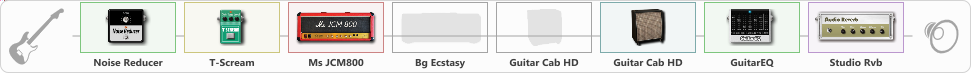# Rammstein Live Aus Berlin 1998

Discussion in 'ToneLib-GFX presets' started by Vallran, Apr 8, 2023.

1. Rammstein Live Aus Berlin 1998

Preset name: Rammstein Live Aus Berlin

Effects chain:Effect: "Noise Reducer" (Dynamics / Filter), active - "yes"
{
"Sens" = 50
"Mode" = Soft
}

Effect: "T-Scream" (Overdrive / Distortion), active - "yes"
{
"Drive" = 20
"Tone" = 83
"Level" = 100
}

Effect: "Ms JCM800" (Amp simulators), active - "yes"
{
"Gain" = 52
"Bass" = 50
"Middle" = 20
"Treble" = 100
"Presence" = 100
"Master" = 100
"Level (dB)" = 1
}

Effect: "Bg Ecstasy" (Amp simulators), active - "no"
{
"Gain" = 32
"Bass" = 68
"Middle" = 32
"Treble" = 100
"Presence" = 100
"Master" = 100
"Level (dB)" = 0
}

Effect: "Guitar Cab HD" (Cabinets), active - "no"
{
"Model" = Bogner Ecstasy (4x12")
"Mic Model" = Dynamic SM57
"Mic Position" = Center
"Mic Distance" = Middle
"Low Cut (Hz)" = 127
"Hi Cut (kHz)" = 20.0
"Mix" = 100
"Level (dB)" = 1
}

Effect: "Guitar Cab HD" (Cabinets), active - "yes"
{
"Model" = Marshall 1960A (4x12")
"Mic Model" = Dynamic SM57
"Mic Position" = Center
"Mic Distance" = Middle
"Low Cut (Hz)" = 123
"Hi Cut (kHz)" = 20.0
"Mix" = 100
"Level (dB)" = 1
}

Effect: "GuitarEQ" (Dynamics / Filter), active - "yes"
{
"160 Hz" = 0
"400 Hz" = 1
"800 Hz" = -2
"1.6 kHz" = 1
"3.2 kHz" = 0
"6.4 kHz" = 2
"12 kHz" = -2
"Level (dB)" = 0
}

Effect: "Studio Rvb" (Reverberation), active - "yes"
{
"Time" = 5.5
"PreDelay" = 2
"LoDamp" = 0
"HiDamp" = 0
"Mix" = 50
}

Note: You will need to download and install the ToneLib-GFX software to use the preset.

File size:
840 bytes
Views:
1,319